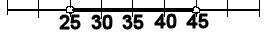### MIDDLE GROUND - Brief Summary of A Confidence Interval: A Range of Expected Values

 A Confidence Interval, A Brief Introduction         A confidence interval is a range of numbers, used to describe a statistical parameter and how sure the statistician is about its value.         This interval might be represented in words, as in:       The average is between 25 and 45.         This interval might be represented in symbols, as in:       25 < µ < 45.         This interval might be represented on a number line, as in:It might be used in conjunction with the area under a normal curve, as in: "within 2 standard deviations of the mean."It might be used when reporting the error in a survey, as in: "65% supported the proposition, with a 4% margin of error."It might be used when completing a two-tailed hypothesis test, as in: "The average is 0. With 95% confidence, test the alternate hypothesis that the average in not 0."In each case the confidence interval is a range of scores.© 2011, 2012 Agnes Azzolino www.mathnstuff.com/math/spoken/here/2class/90/confid.htm